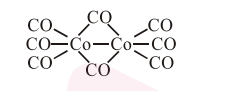# The number of bridging`
Question:

The number of bridging $\mathrm{CO}$ ligand (s) and $\mathrm{Co}-$ Co bond (s) in $\mathrm{CO}_{2}(\mathrm{CO}) \mathrm{g}$, respectively are :-

1. 0 and 2

2. 2 and 0

3. 4 and 0

4. 2 and 1

Correct Option: , 4

Solution:Bridging $\mathrm{CO}$ are 2 and $\mathrm{Co}-\mathrm{Co}$ bond is 1 .